# Field-Oriented Control of PMSM Using Reinforcement Learning

This example shows you how to use the control design method of reinforcement learning to implement field-oriented control (FOC) of a permanent magnet synchronous motor (PMSM). The example uses FOC principles. However, it uses the reinforcement learning (RL) agent instead of the PI controllers. For more details about FOC, see Field-Oriented Control (FOC).

This figure shows the FOC architecture with the reinforcement learning agent. For more details about the reinforcement learning agents, see Reinforcement Learning Agents (Reinforcement Learning Toolbox).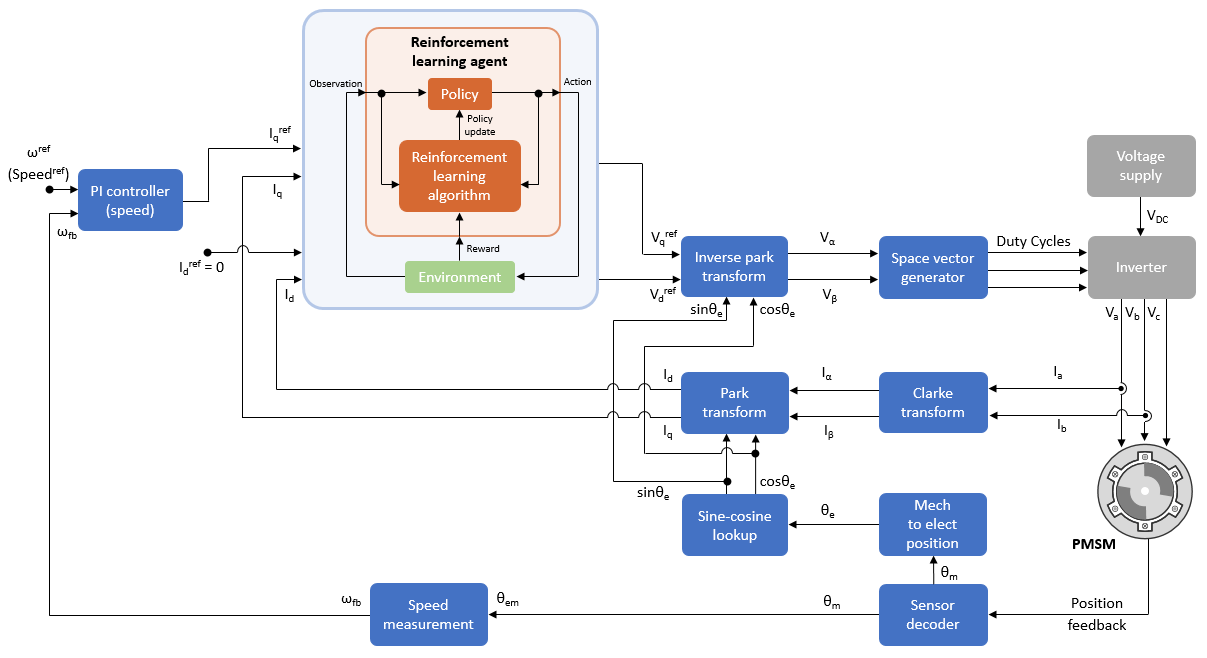The reinforcement learning agent regulates the `d`-axis and `q`-axis currents and generates the corresponding stator voltages that drive the motor at the required speed.

The speed-tracking performance of an FOC algorithm that uses a reinforcement learning agent is similar to that of a PI-controller-based FOC.

### Model

The example includes the mcb_pmsm_foc_sim_RL model.

Note: You can use this model only for simulation.

This model includes the FOC architetcure that uses the reinforcement learning agent. You can use the openExample command to open the Simulink® model.

`openExample('mcb/FieldOrientedControlOfPMSMUsingReinforcementLearningExample','supportingFile','mcb_pmsm_foc_sim_RL.slx');`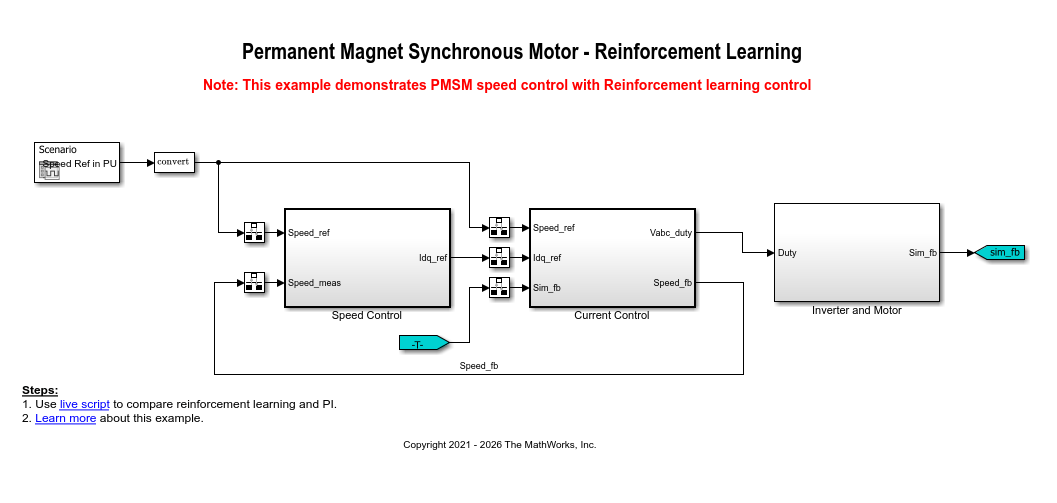When you open the model, it loads the configured parameters including the motor parameters to the workspace for simulation. To view and update these parameters, open the `mcb_pmsm_foc_sim_RL_data.m` model initialization script file. For details about the control parameters and variables available in this script, see Estimate Control Gains and Use Utility Functions.

You can access the reinforcement learning setup available inside the Current Controller Systems subsystem by running this command.

`open_system('mcb_pmsm_foc_sim_RL/Current Control/Control_System/Closed Loop Control/Current Controller Systems');`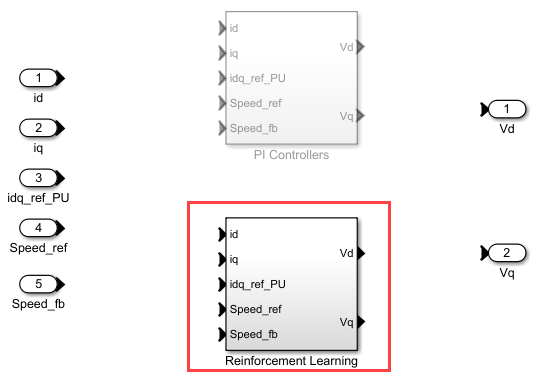For more information about setting up and training a reinforcement learning agent to control a PMSM, see Train TD3 Agent for PMSM Control (Reinforcement Learning Toolbox).

Note:

• Training a reinforcement learning agent is a computationally intensive process that may take several hours to complete.

• The agent in this example was trained using the PWM frequency of 5 KHz. Therefore, the model uses this frequency by default. To change this value, train the reinforcement learning agent again by using a different PWM frequency and update the `PWM_frequency` variable in the `mcb_pmsm_foc_sim_RL_data.m` model initialization script. You can use the following command to open the model initialization script.

`edit mcb_pmsm_foc_sim_RL_data;`

### Required Mathworks® Products

• Motor Control Blockset™

• Reinforcement Learning Toolbox™

### Simulate Model

Follow these steps to simulate the model.

1. Click mcb_pmsm_foc_sim_RL to open the model included with this example.

2. Run this command to select the Reinforcement Learning variant of the Current Controller Systems subsystem available inside the FOC architecture.

`ControllerVariant='RL';`

You can navigate to the Current Controller Systems subsystem to verify if the Reinforcement Learning subsystem variant is active.

`open_system('mcb_pmsm_foc_sim_RL/Current Control/Control_System/Closed Loop Control/Current Controller Systems');`Note: The model selects the Reinforcement Learning subsystem variant by default.

3. Run this command to load the pre-trained reinforcement learning agent.

`load('rlPMSMAgent.mat');`

Note: The reinforcement learning agent in this example was trained to use the speed references of 0.2, 0.4, 0.6, and 0.8 PU (per-unit). For information related to the per-unit system, see Per-Unit System.

4. Click Run on the Simulation tab to simulate the model. You can also run this command to simulate the model.

`sim('mcb_pmsm_foc_sim_RL.slx');`
```### The Lq is observed to be lower than Ld. ### ### Using the lower of these two for the Ld (internal variable) ### ### and higher of these two for the Lq (internal variable) for computations. ### ### The Lq is observed to be lower than Ld. ### ### Using the lower of these two for the Ld (internal variable) ### ### and higher of these two for the Lq (internal variable) for computations. ### ```

5. Click Data Inspector on the Simulation tab to open the Simulation Data Inspector. Select one or more of these signals to observe and analyze the simlation results related to speed tracking and controller performance.

• `Speed_ref`

• `Speed_fb`

• `iq_ref`

• `iq`

• `id_ref`

• `id`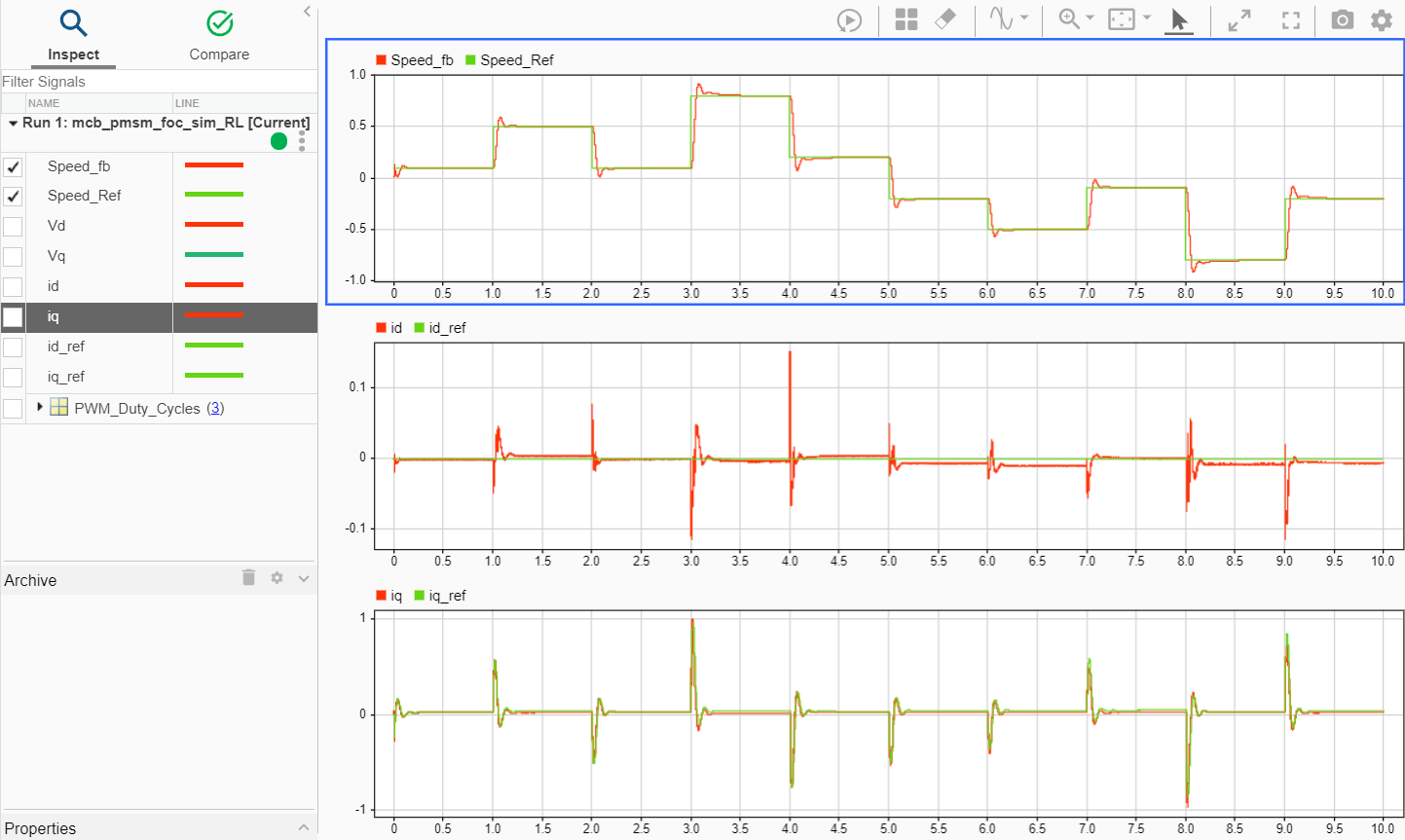In the preceding example:

• The combination of PI and reinforcement learning controllers achieve the required speed by tracking the changes to the speed reference signal.

• The second and third data inspector plots show that the trained reinforcement learning agent acts as a current controller and successfully tracks both the `Id` and `Iq` reference currents. However, a small steady state error exists between the reference and acutal values of `Id` and `Iq` currents.

### Use Simulation to Compare RL Agent with PI Controllers

Use these steps to analyze the speed tracking and controller performance of PI controllers and compare them with that of reinforcement learning agent:

1. Click mcb_pmsm_foc_sim_RL to open the model included with this example.

2. Run this command to select the PI Controllers variant of the Current Controller Systems subsystem available inside the FOC architecture.

`ControllerVariant='PI';`

You can navigate to the Current Controller Systems subsystem to verify if the PI Controllers subsystem variant is active.

`open_system('mcb_pmsm_foc_sim_RL/Current Control/Control_System/Closed Loop Control/Current Controller Systems');`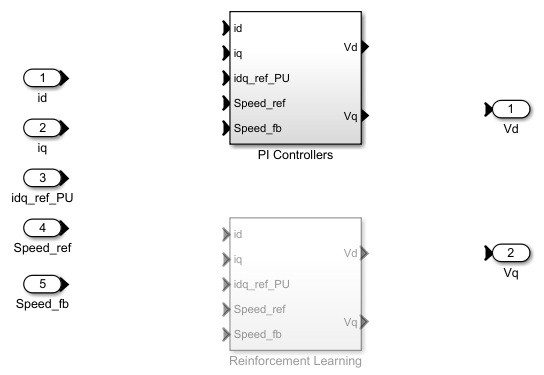NOTE: The model selects the Reinforcement Learning subsystem variant by default.

3. Click Run on the Simulation tab to simulate the model. You can also run this command to simulate the model.

`sim('mcb_pmsm_foc_sim_RL.slx');`
```### The Lq is observed to be lower than Ld. ### ### Using the lower of these two for the Ld (internal variable) ### ### and higher of these two for the Lq (internal variable) for computations. ### ### The Lq is observed to be lower than Ld. ### ### Using the lower of these two for the Ld (internal variable) ### ### and higher of these two for the Lq (internal variable) for computations. ### ```

4. Click Data Inspector on the Simulation tab to open the Simulation Data Inspector. Select one or more of these signals to observe and analyze the simlation results related to speed tracking and controller performance.

• `Speed_ref`

• `Speed_fb`

• `iq_ref`

• `iq`

• `id_ref`

• `id`

5. Compare these results with the previous simulation run results obtained by using the RLAgent (Reinforcement Learning) subsystem variant.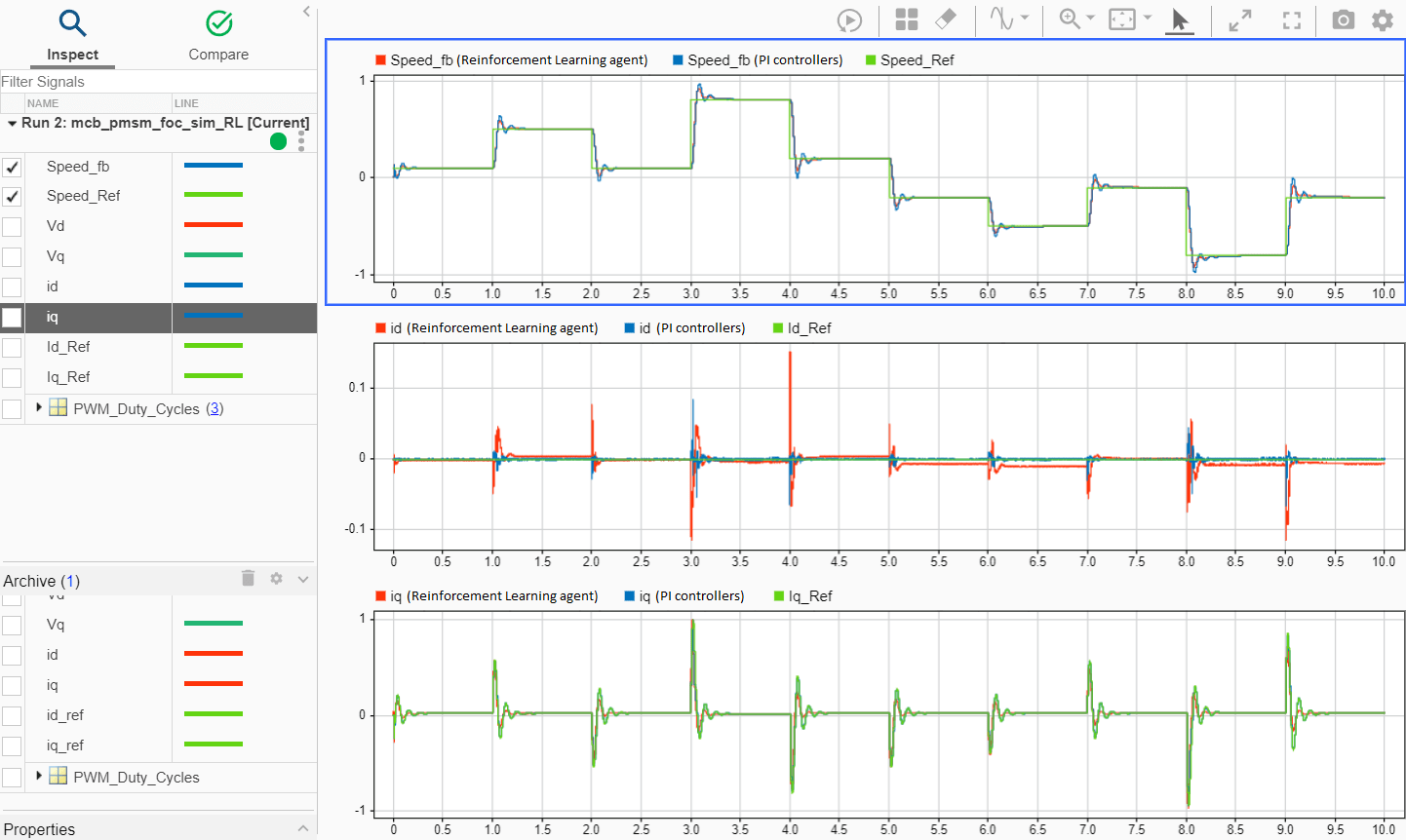In the preceding example:

• The red signals show the simulation results that you obtain using the RLAgent (Reinforcement Learning) subsystem variant.

• The blue signals show the simulation results that you obtain using the PIControllers (PI Controllers) subsystem variant.

• The plots indicate that (with an exception of `Id` reference current tracking) the performance of reinforcement learning agent is similar to the PI controllers. You can improve the current tracking performance of the reinforcement learning agent by further training the agent and tuning the hyperparameters.

NOTE: You can also update the reference speed to higher values and similarly compare the performances between reinforcement learning agent and PI controllers.